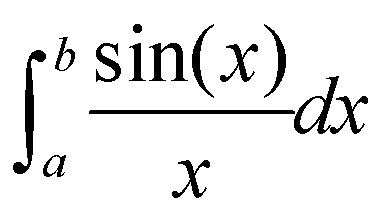# csu 1806 & csu 1742 (simpson公式+最短路)

## 1806: Toll

Time Limit: 5 Sec  Memory Limit: 128 MB  Special Judge
Submit: 256  Solved: 74
[Submit][Status][Web Board]

## Description

In ICPCCamp, there are n cities and m unidirectional roads between cities. The i-th road goes from the ai-th city to the bi-th city. For each pair of cities u and v, there is at most one road from u to v.
As traffic in ICPCCamp is becoming heavier, toll of the roads also varies. At time t, one should pay (ci⋅t+di) dollars to travel along the i-th road.
Bobo living in the 1-st city would like to go to the n-th city. He wants to know the average money he must spend at least if he starts from city 1 at t∈[0,T]. Note that since Bobo's car is super-fast, traveling on the roads costs him no time.
Formally, if f(t) is the minimum money he should pay from city 1 to city n at time t, Bobo would like to find## Input

The first line contains 3 integers n,m,T (2≤n≤10,1≤m≤n(n-1),1≤T≤104).
The i-th of the following m lines contains 4 integers ai,bi,ci,di (1≤ai,bi≤n,ai≠bi,0≤ci,di≤103).
It is guaranteed that Bobo is able to drive from city 1 to city n.

## Output

A floating number denotes the answer. It will be considered correct if its absolute or relative error does not exceed 10-6.

## Sample Input

3 3 2
1 2 1 0
2 3 1 0
1 3 1 1
3 3 2
1 2 1 0
2 3 1 0
1 3 0 5


## Sample Output

1.75000000
2.00000000

#include <bits/stdc++.h>
using namespace std;
typedef long long LL;
const double INF = 999999999;
struct Edge{
int v,next;
int c,d;
}edge;
int n,m,T;
void init(){
tot = 0;
}
void addEdge(int u,int v,int c,int d,int &k){
}
double dis;
bool vis;
double F(double x){
for(int i=1;i<=n;i++){
dis[i] = INF;
vis[i] = false;
}
dis = 0;
queue<int> q;
q.push(1);
while(!q.empty()){
int u = q.front();
q.pop();
vis[u] = false;
for(int k = head[u];k!=-1;k = edge[k].next){
int v = edge[k].v,c = edge[k].c,d = edge[k].d;
double t = x*c+d;
if(dis[v]>dis[u]+t){
dis[v] = dis[u]+t;
if(!vis[v]){
vis[v] = true;
q.push(v);
}
}
}
}
double ret = dis[n];
return ret;
}
// 三点simpson法。这里要求F是一个全局函数
double simpson(double a,double b){
double c =  a+(b-a)/2;
return (F(a) + 4*F(c) + F(b))*(b-a)/6;
}
// 自适应Simpson公式（递归过程）。已知整个区间[a,b]上的三点simpson值A
double asr(double a , double b ,double eps ,double A){
double c = a+ (b-a)/2;
double L = simpson(a,c) ,R = simpson(c,b);
if(fabs(A-L-R)<=15*eps) return L + R +(A-L-R)/15;
return asr(a,c,eps/2,L) + asr(c,b,eps/2,R);
}
// 自适应Simpson公式（主过程）
double asr(double a, double b, double eps) {
return asr(a, b, eps, simpson(a, b));
}

int main()
{
while(scanf("%d%d%d",&n,&m,&T)!=EOF){
init();
for(int i=0;i<m;i++){
int u,v,c,d;
scanf("%d%d%d%d",&u,&v,&c,&d);
}
double ans = asr(0,T,1e-6)/T;
printf("%.8lf\n",ans);
}
return 0;
}

csu 1742

## 1742: Integral Function

Time Limit: 1 Sec  Memory Limit: 128 MB
Submit: 79  Solved: 27
[Submit][Status][Web Board]

## Description

In mathematics, several function are unable to integral. For example:But you can get the answer by computer.

## Input

There are no more than T (T<=30) cases. Each case include two integer a, b (0<a <= b<=10).

## Sample Input

1 1
1 2
2 8

## Sample Output

0
6593
-312这题更好用..直接带进去算
#include <bits/stdc++.h>
using namespace std;
typedef long long LL;

double F(double x){
return sin(x)/x;
}
// 三点simpson法。这里要求F是一个全局函数
double simpson(double a,double b){
double c =  a+(b-a)/2;
return (F(a) + 4*F(c) + F(b))*(b-a)/6;
}
// 自适应Simpson公式（递归过程）。已知整个区间[a,b]上的三点simpson值A
double asr(double a , double b ,double eps ,double A){
double c = a+ (b-a)/2;
double L = simpson(a,c) ,R = simpson(c,b);
if(fabs(A-L-R)<=15*eps) return L + R +(A-L-R)/15;
return asr(a,c,eps/2,L) + asr(c,b,eps/2,R);
}
// 自适应Simpson公式（主过程）
double asr(double a, double b, double eps) {
return asr(a, b, eps, simpson(a, b));
}

int main()
{
double a,b;
while(scanf("%lf%lf",&a,&b)!=EOF){
printf("%d\n",(int)(asr(a,b,1e-6)*10000));
}
return 0;
}



posted @ 2016-09-29 17:47  樱花庄的龙之介大人  阅读(...)  评论(...编辑  收藏